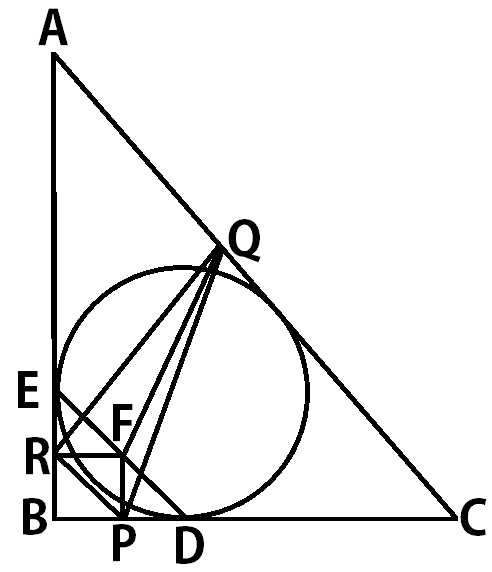# The Midpoint Of The Points Of Tangency

Geometry Level 5In right angled $\triangle ABC$, $\angle ABC= 90°$ and $\angle BCA = 60°$. The incircle of $\triangle ABC$ touches sides $\overline{BC}$ and $\overline{AB}$ at points $D$ and $E$ respectively. Let $F$ be the midpoint of $DE$. $P, Q, R$ are the feet of perpendiculars from $F$ on $BC, CA, AB$ respectively. Let $k= \dfrac{AB+BC+CA}{PQ+QR+RP}$. Find $\left \lfloor 100k \right \rfloor$.

Details and assumptions

• $\lfloor x \rfloor$ denotes the greatest integer function, i.e. it is the greatest integer not exceeding $x$. For example, $\lfloor 2.3 \rfloor = 2, \lfloor \pi \rfloor = 3, \lfloor 5 \rfloor= 5.$

• Once you find the exact form of $k$, you might use a calculator to proceed.

• The last digit of the answer that is being accepted is $8$. If your answer slightly differs from the intended one because of approximation errors, you should enter the closest integer whose last digit is $8$.

• GeoGebra users will be prosecuted.

×

Problem Loading...

Note Loading...

Set Loading...